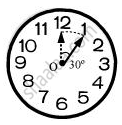Share

# The length of the minute hand of a clock is 14 cm. Find the area swept by the minute hand in 5 minutes. - CBSE Class 10 - Mathematics

ConceptAreas of Sector and Segment of a Circle

#### Question

The length of the minute hand of a clock is 14 cm. Find the area swept by the minute hand in 5 minutes.  [Use Π = 22/7]

#### SolutionWe know that in 1 hour (i.e., 60 minutes), the minute hand rotates 360°.

In 5 minutes, minute hand will rotate = 360^@/60xx5 = 30^@

Therefore, the area swept by the minute hand in 5 minutes will be the area of a sector of 30° in a circle of 14 cm radius.

Area of sector of angle θ = θ/(360°) xx pir^2

Area of sector of 30° =(30°)/(360°)xx22/7xx14xx14

=22/12xx2xx14

=(11xx14)/3

=154/3 cm2

Therefore, the area swept by the minute hand in 5 minutes is 154/3 cm2

Is there an error in this question or solution?

#### APPEARS IN

NCERT Solution for Mathematics Textbook for Class 10 (2019 to Current)
Chapter 12: Areas Related to Circles
Ex. 12.20 | Q: 3 | Page no. 230
Solution The length of the minute hand of a clock is 14 cm. Find the area swept by the minute hand in 5 minutes. Concept: Areas of Sector and Segment of a Circle.
S